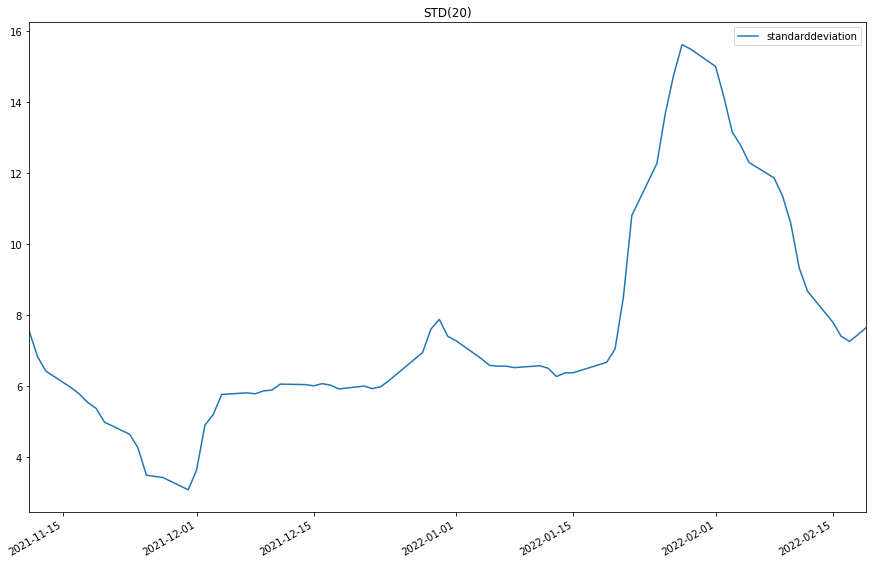# Indicator Reference

## Standard Deviation

### Introduction

This indicator computes the n-period population standard deviation.

### Create Manual Indicators

You can manually create a StandardDeviation indicator, so it doesn’t automatically update. Manual indicators let you update their values with any data you choose. The following reference table describes the StandardDeviation constructor.

### StandardDeviation()1/2

            StandardDeviation QuantConnect.Indicators.StandardDeviation (
int  period
)


On a data set of size N will use an N normalizer and would thus be biased if applied to a subset.

### StandardDeviation()2/2

            StandardDeviation QuantConnect.Indicators.StandardDeviation (
string  name,
int     period
)


On a data set of size N will use an N normalizer and would thus be biased if applied to a subset.

### Update Manual Indicators

You can update the indicator automatically or manually.

#### Automatic Update

To register a manual indicator for automatic updates with the security data, call the RegisterIndicator method.


private StandardDeviation _std;

// In Initialize()
_std = new StandardDeviation(name, period);
_std.Updated += IndicatorUpdateMethod;

RegisterIndicator(symbol, _std, Resolution.Daily);

// In IndicatorUpdateMethod()
{
var indicatorValue = _std.Current.Value;
}

# In Initialize()
self.std = StandardDeviation(name, period)
self.std.Updated += self.IndicatorUpdateMethod

self.RegisterIndicator(symbol, self.std, Resolution.Daily)

# In IndicatorUpdateMethod()
indicator_value = self.std.Current.Value

To customize the data that automatically updates the indicator, see Custom Indicator Periods and Custom Indicator Values.

#### Manual Update

Updating your indicator manually enables you to control when the indicator is updated and what data you use to update it. To manually update the indicator, call the Update method with time/decimal pair. The indicator will only be ready after you prime it with enough data.


private StandardDeviation _std;
private Symbol symbol;

// In Initialize()
_std = new StandardDeviation(period);

// In OnData()
if (data.ContainsKey(_symbol))
{
_std.Update(data[symbol].EndTime, data[symbol].High);
}
{
var indicatorValue = _std.Current.Value;
}

# In Initialize()
self.std = StandardDeviation(period)

# In OnData()
if data.ContainsKey(self.symbol):
self.std.Update(data[self.symbol].EndTime, data[self.symbol].High)
indicator_value = self.std.Current.Value

### Create Automatic Indicators

The STD method creates an StandardDeviation indicator, sets up a consolidator to update the indicator, and then returns the indicator so you can use it in your algorithm.

The following reference table describes the STD method:

### STD()1/1

            StandardDeviation QuantConnect.Algorithm.QCAlgorithm.STD (
Symbol                           symbol,
Int32                            period,
*Nullable<Resolution>      resolution,
*Func<IBaseData, Decimal>  selector
)


Creates a new StandardDeviation indicator. This will return the population standard deviation of samples over the specified period.

If you don't provide a resolution, it defauls to the security resolution. If you provide a resolution, it must be greater than or equal to the resolution of the security. For instance, if you subscribe to hourly data for a security, you should update its indicator with data that spans 1 hour or longer.

### Get Indicator Values

To get the value of the indicator, use its Current.Value attribute.

private StandardDeviation _std;

// In Initialize()
_std = STD(symbol, period);

// In OnData()
{
var current = _std.Current.Value;
}
# In Initialize()
self.std = self.STD(symbol, period)

# In OnData()
current = self.std.Current.Value


### Visualization

To plot indicator values, in the OnData event handler, call the Plot method.

private StandardDeviation _std;

// In Initialize()
_std = STD(symbol, period);

// In OnData()
{
Plot("My Indicators", "standarddeviation", _std.Current);
}
# In Initialize()